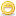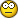1. ##Delivered Engine Torque

I have been scanning delivered torque for calibrating my shift speed changes and I have not seen the torque much above 150 Nm.

When using this to calculate flywheel torque the best I can get is 140kw.

This seems to be less than what I expected.

I have 221 rwkw.

Any thoughts?Reply With Quote

2. ##In the software, there is a bug with vehicle weight and how it stores the value in kg/lbs.

Try changing the vehicle's weight and then go back and look at what its stored value is...Reply With Quote

3. ##Re: Delivered Engine TorqueOriginally Posted by daveb
I have been scanning delivered torque for calibrating my shift speed changes and I have not seen the torque much above 150 Nm.

When using this to calculate flywheel torque the best I can get is 140kw.

This seems to be less than what I expected.

I have 221 rwkw.

Any thoughts?
DaveB,

You don't happen to be driving a Ute do you??.
You can pretty much ignore that value on Utes, GMH screwed the cals to use that Torque figure for other things (like slowing the F.Pump down at WOT).
Using the navigator in the tuner take a look at parameter number 'B1904'.

Cheers,
RossReply With Quote

4. ##Thanks guys,

Power = Torque * angular velocity

So if we know these values we can calculate Power without knowing the weight.

From memory:

Angular velocity is measured in radians per second (and there is 2 pi radians in a full rotation – ie one rpm). If torque is measured in Nm then Power will be in kw.

So flywheel power should be = torque*angular velocity

Where torque is {GM.TRQENG} ie deliver engine torque

and

angular velocity is 2*pi*rpm/60 as angular velocity = 0.1047*rpm

Or rpm/9.549.

So Power = GM.TRQENG*rpm/9.949

Where power will be in watts so divide by 1000 to convert to kilowatts kw. Which is:

P = TRQENG*rpm/9949 or TRQENG*rpm/9950

Or HP = ({GM.TRQENG.ftlb}*{SAE.RPM.rpm})/5252 (accounting for the difference in hp and kw)

So vehicle weight is not a factor in this equation, but the torque reading must be correct for it to work.

BTW vehicle weight is critical to calculate net Power at the wheels calculations. Another way to look at Power is based on Newton’s laws.

Work done = Force*distance (where distance is the distance the force was applied over).

Power is the rate of doing work (ie how much work you are doing in any given time). So;
Power = Force*velocity (as power is the rate of work done and velocity is the rate of distance traveled )

Newtons 2nd law says:

Force = mass*acceleration

So Power = mass*acceleration*velocity (where velocity is in meters per second and acceleration in meters per second per second)

acceleration = rate of change in velocity; this is calculated by the function dx({SAE.VSS.kph},6) – the 6 is the number of samples over which the rate of change is calculated ) – NB I am guessing about this so please correct me if I am wrong..

A meter per second is 3.6 kph (ie 10 meters per second is 36 kph so we must divide kph by 3.6 to convert into meters per second).

So Power (in kw) at the wheels calc will be

Power = gvmkg()*{SAE.VSS.kph}/3.6*dx{SAE.VSS.kph}/3.6

= gvmkg()*{SAE.VSS.kph}/*dx{SAE.VSS.kph}/(3.6*3.6)

= gvmkg()*{SAE.VSS.kph}/*dx{SAE.VSS.kph}/(12.96)

To convert into kw we divide by another 1000:

Power = gvmkg()*{SAE.VSS.kph}/*dx{SAE.VSS.kph}/12960

This equates to

Power = HP = gvmlb()*{SAE.VSS.mph}*dx({SAE.VSS.mph},6)/8226.63 (accounting for the difference in hp and kw and kg and lbs)

NB this calculation is based on the power pushing the car forward which is really the power at the wheels minus the air resistance power (air resistance * velocity). Air resistance increases proportional to the square of the velocity and therefore power loss due to air resistance is proportional to the cube of the speed of the vehicle.

So while we are at it, this is why, quarter times are inversely proportional to the cube of the power of the car (for a given mass and air drag coefficient). So doubling your power (given all other thing equal) will reduce quarter time by approx 21% (eg 13 sec to 10.3 sec). In other words the 10 sec club is not cheap :? ;.

I have skipped over a number of things here, so feel free to ask for more details if yhou are interested.

Ross:

My car is a VTII Calais sedan. I will check out the parameter 'B1904'. But I do not know why the torque values are about half what they should be.

Thanks,Reply With Quote

5. ##The EFILive software uses the vehicle's weight to calculate RWHP. This calculated hp is much lower than it should be. I've tracked these low values down to a bug in how EFILive stores the vehicle's weight.

Unfortunately, I haven't had time to get the details to Paul yet so he can fix it. When this bug is fixed, I believe the hp/tq numbers will be more in line with what is expected.

We are talking about two different problems here with your torque problem and my hp problem but they are a related topic.Reply With Quote

6. ##Reply With Quote

7. ##Originally Posted by SinisterSS
The EFILive software uses the vehicle's weight to calculate RWHP. This calculated hp is much lower than it should be. I've tracked these low values down to a bug in how EFILive stores the vehicle's weight.
I have seen the samething... it seems to me that the GVW in lb gets converted to kg everytime you access it, or something like that, so it gets smaller each time.Reply With Quote

8. ##Joecar, please email your sae_generic file with that calculated PID.Reply With Quote

9. ##Originally Posted by SinisterSS
Joecar, please email your sae_generic file with that calculated PID.Successfully added my first custom PID tonight. Thanks Joecar! 8)

Here's the data logged from today: supercharged LS6 - 800+ IRHP.Played around with the dx constant but it didn't change it by very much. It's high by about 2x the actual I'd say. Got the right weight in there so I'm pretty much scratching my head at this point. :?:Reply With Quote

10. ##Any further thoughts on the delivered engine torque, this is still a mystery.Reply With Quote

####Posting Permissions

• You may not post new threads
• You may not post replies
• You may not post attachments
• You may not edit your posts
•